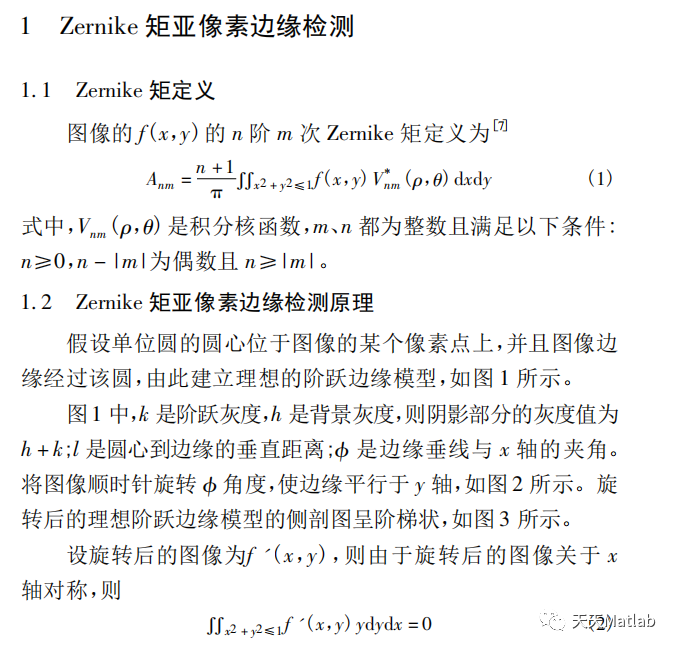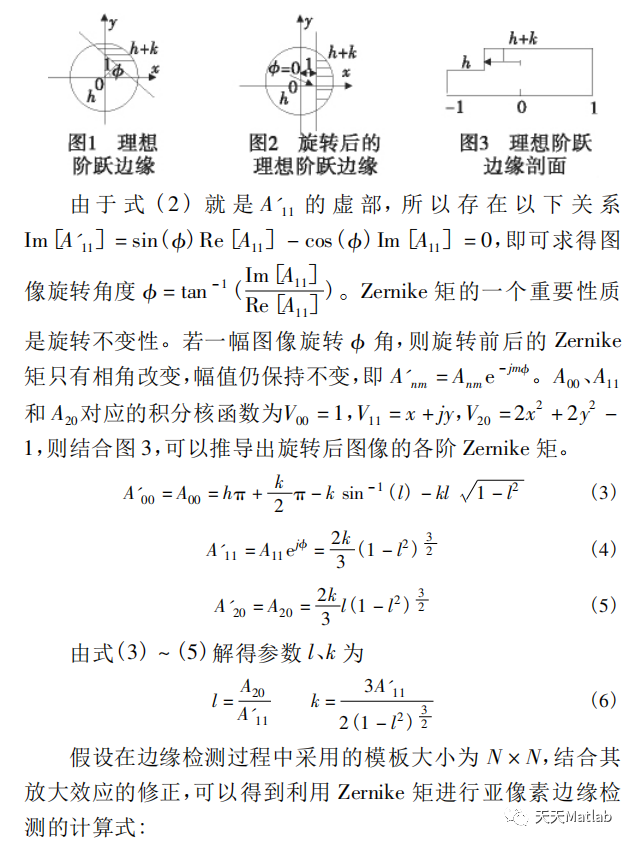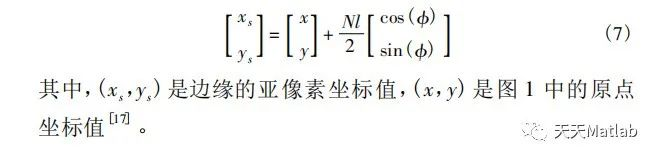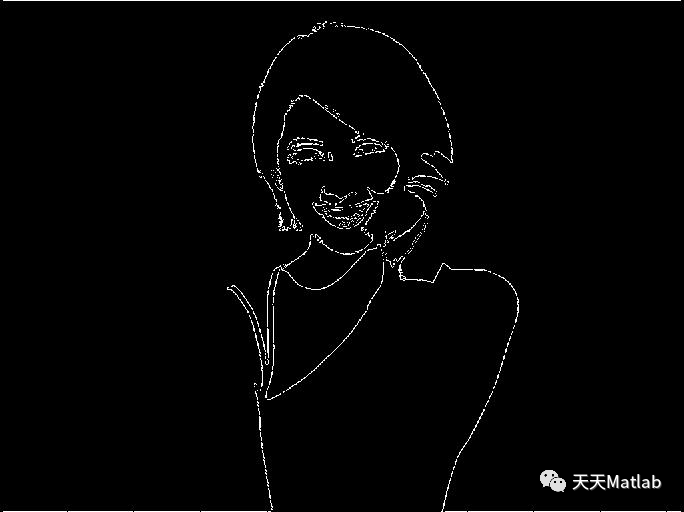• 亚像素边缘检测提取算法的实现
千次阅读
2021-11-29 00:04:27
       亚像素边缘检测提取算法的实现
其中包括插值法、拟合法、基于灰度矩法、基于zernike矩法等


通过Matlab软件运用zernike矩的检测算法
通过如下代码可实现
function zernike7(I)

% 7*7Zernike模板
M00=…
[
0 0.0287 0.0686 0.0807 0.0686 0.0287 0
0.0287 0.0815 0.0816 0.0816 0.0816 0.0815 0.0287
0.0686 0.0816 0.0816 0.0816 0.0816 0.0816 0.0686
0.0807 0.0816 0.0816 0.0816 0.0816 0.0816 0.0807
0.0686 0.0816 0.0816 0.0816 0.0816 0.0816 0.0686
0.0287 0.0815 0.0816 0.0816 0.0816 0.0815 0.0287
0 0.0287 0.0686 0.0807 0.0686 0.0287 0
];
M11R=…
[
0 -0.015 -0.019 0 0.019 0.015 0
-0.0224 -0.0466 -0.0233 0 0.0233 0.0466 0.0224
-0.0573 -0.0466 -0.0233 0 0.0233 0.0466 0.0573
-0.069 -0.0466 -0.0233 0 0.0233 0.0466 0.069
-0.0573 -0.0466 -0.0233 0 0.0233 0.0466 0.0573
-0.0224 -0.0466 -0.0233 0 0.0233 0.0466 0.0224
0 -0.015 -0.019 0 0.019 0.015 0
];
M11I=…
[
0 -0.0224 -0.0573 -0.069 -0.0573 -0.0224 0
-0.015 -0.0466 -0.0466 -0.0466 -0.0466 -0.0466 -0.015
-0.019 -0.0233 -0.0233 -0.0233 -0.0233 -0.0233 -0.019
0 0 0 0 0 0 0
0.019 0.0233 0.0233 0.0233 0.0233 0.0233 0.019
0.015 0.0466 0.0466 0.0466 0.0466 0.0466 0.015
0 0.0224 0.0573 0.069 0.0573 0.0224 0
];
M20=…
[
0 0.0225 0.0394 0.0396 0.0394 0.0225 0
0.0225 0.0271 -0.0128 -0.0261 -0.0128 0.0271 0.0225
0.0394 -0.0128 -0.0528 -0.0661 -0.0528 -0.0128 0.0394
0.0396 -0.0261 -0.0661 -0.0794 -0.0661 -0.0261 0.0396
0.0394 -0.0128 -0.0528 -0.0661 -0.0528 -0.0128 0.0394
0.0225 0.0271 -0.0128 -0.0261 -0.0128 0.0271 0.0225
0 0.0225 0.0394 0.0396 0.0394 0.0225 0
];

if length(size(I))==3 I=rgb2gray(I); end
I=im2bw(I,0.6);
K=double(I);
[m n]=size(K);
xs=double(zeros(m,n));
ys=double(zeros(m,n));

% 卷积运算
A11I=conv2(M11I,K);
A11R=conv2(M11R,K);
A20=conv2(M20,K);

% 截掉多余部分
A11I=A11I(4:end-3,4:end-3);
A11R=A11R(4:end-3,4:end-3);
A20=A20(4:end-3,4:end-3);

J=zeros(size(K));
boundary=J;
theta=atan2(A11I,A11R);%计算theta

%计算边缘的三个参数
A11C=A11R.*cos(theta)+A11I.sin(theta);
l=A20./A11C;
k=1.5
A11C./((1-l.2).1.5);
e=abs(l)>1/3.5;
k(e)=0;

%边缘判断条件
a=abs(l)<1/sqrt(2)*2/7;
b=abs(k)>max(I(😃)/10;
% a,b分别为距离和边缘强度判断结果
J(a&b)=1;
%将图像的最边缘去除
% boundary(2:end-1,2:end-1)=1;
% J(~boundary)=0;
format short
% [x,y]=find(J1);%边缘的像素级坐标
% O=[x y];
% Z=[x+l(find(J
1)).*cos(theta(find(J1))) y+l(find(J1)).*sin(theta(find(J==1)))];%亚像素坐标
% % fprintf(’%.4f %.4f\n’,Z’);

[L,num]=bwlabel(J,8);%对二值图像进行标记
%自动化搜索连通域
s=zeros(1,num);
for i=1:num
s(i)=size(find(L==i),1);
end
[bwL,label]=sort(s,‘descend’);
if label(1)<label(2)
index1=label(1);
index2=label(2);
else
index1=label(2);
index2=label(1);
end

%计算左边探针的最前端坐标
[r1,c1]=find(L==index1);
A1=[r1 c1];
y1=max(A1(:,2));%该连通域中y最大值为针尖处
x1=max(A1(find(A1(:,2)==y1),1));
x1sub=x1+3.5*l(x1,y1)cos(theta(x1,y1));
y1sub=y1+3.5
l(x1,y1)*sin(theta(x1,y1));

%计算最右边探针的最前端坐标
[r2,c2]=find(L==index2);
A2=[r2 c2];
y2=min(A2(:,2));%该连通域中y最小为连通域
x2=max(A2(find(A2(:,2)==y2),1));
x2sub=x2+3.5*l(x2,y2)cos(theta(x2,y2));
y2sub=y2+3.5
l(x2,y2)*sin(theta(x2,y2));

% [x1sub y1sub],[x2sub,y2sub]
subplot(221)
imshow(J)
% figure;
% imcontour(J,1)

% 边界提取
% subplot(122);
% bwimg = bwmorph(J,‘remove’);
% imshow(bwimg)

subplot(222);
I41=imfill(J,‘holes’);
imshow(I41)
title(‘孔洞填充图像’);
% 提取最外围边缘
subplot(223);
I4=bwperim(I41);
imshow(I4); title(‘边缘图像’);
% 去除面积小于150px物体
subplot(224);
I5=bwareaopen(I4,100);
imshow(I5);

更多相关内容
• 执行快速准确的亚像素边缘检测，基于改进的zernike矩方法，有需要的可以下载~~
• 一种快速亚像素边缘检测算法的实现的理论，可以快速实现像素的算法，在时间上有一定的优势，先看理论，然后自己通过理论用C++实现
• 1. 用MATLAB实现的基于Zernike矩的亚像素边缘检测算法，有图片实例，一键运行出结果 2. 包含理论资料，在本人博客也有介绍https://betterbench.blog.csdn.net/article/details/121612652
• 本压缩包包括一个亚像素边缘检测代码，以及一个测试图片，从像素的角度去解决边缘检测问题，并且与Canny算子做比较，效果显著！
• 利用opencv的亚像素级别的边缘检测和获取，添加了原有程序的包含文件和库，可以直接调试运行，个边参数可以根据实际情形修改
• 本程序使用Zernike矩来进行边缘像素检测，并添加了一个例子，欢迎需要亚像素边缘检测的同学讨论。
• 亚像素边缘检测的matlab代码，比一般的边缘检测代码精确matlab
• 修改过的亚像素边缘检测修改过的亚像素边缘检测修改过的亚像素边缘检测
• 针对传统算子定位精度较低,检测出的边缘较粗的缺点,本文提出了基于Zernike矩的亚像素边缘检测算子,并推导出 的模板系数.该算法采用积分算子的形式对噪声不敏感,能有效的抑制噪声,较好的提取出图像边缘并实现较高的...

## 1 简介

针对传统算子定位精度较低,检测出的边缘较粗的缺点,本文提出了基于Zernike矩的亚像素边缘检测算子,并推导出 的模板系数.该算法采用积分算子的形式对噪声不敏感,能有效的抑制噪声,较好的提取出图像边缘并实现较高的定位,有效克服了传统算子如Sobel算子进行边缘检测时检测的边缘较粗,误差较大,以及对噪声敏感的缺点.实验结果证明,相比Sobel算子Zernike矩算子能够实现精确定位,并且其边缘检测精度能够达到亚像素级.## 2 部分代码

function zernike7(I)

% 7*7Zernike模板

M00=...

[

0 0.0287 0.0686 0.0807 0.0686 0.0287 0

0.0287 0.0815 0.0816 0.0816 0.0816 0.0815 0.0287

0.0686 0.0816 0.0816 0.0816 0.0816 0.0816 0.0686

0.0807 0.0816 0.0816 0.0816 0.0816 0.0816 0.0807

0.0686 0.0816 0.0816 0.0816 0.0816 0.0816 0.0686

0.0287 0.0815 0.0816 0.0816 0.0816 0.0815 0.0287

0 0.0287 0.0686 0.0807 0.0686 0.0287 0

];

M11R=...

[

0 -0.015 -0.019 0 0.019 0.015   0

-0.0224 -0.0466 -0.0233 0 0.0233 0.0466 0.0224

-0.0573 -0.0466 -0.0233 0 0.0233 0.0466 0.0573

-0.069 -0.0466 -0.0233 0 0.0233 0.0466 0.069

-0.0573 -0.0466 -0.0233 0 0.0233 0.0466 0.0573

-0.0224 -0.0466 -0.0233 0 0.0233 0.0466 0.0224

0 -0.015 -0.019 0 0.019 0.015   0

];

M11I=...

[

0 -0.0224 -0.0573 -0.069 -0.0573 -0.0224   0

-0.015 -0.0466 -0.0466 -0.0466 -0.0466 -0.0466 -0.015

-0.019 -0.0233 -0.0233 -0.0233 -0.0233 -0.0233 -0.019

0    0    0    0    0    0   0

0.019 0.0233 0.0233 0.0233 0.0233 0.0233 0.019

0.015 0.0466 0.0466 0.0466 0.0466 0.0466 0.015

0 0.0224 0.0573 0.069 0.0573 0.0224   0

];

M20=...

[

0 0.0225 0.0394 0.0396 0.0394 0.0225 0

0.0225 0.0271 -0.0128 -0.0261 -0.0128 0.0271 0.0225

0.0394 -0.0128 -0.0528 -0.0661 -0.0528 -0.0128 0.0394

0.0396 -0.0261 -0.0661 -0.0794 -0.0661 -0.0261 0.0396

0.0394 -0.0128 -0.0528 -0.0661 -0.0528 -0.0128 0.0394

0.0225 0.0271 -0.0128 -0.0261 -0.0128 0.0271 0.0225

0 0.0225 0.0394 0.0396 0.0394 0.0225 0

];

if length(size(I))==3 I=rgb2gray(I); end

I=im2bw(I,0.6);

K=double(I);

[m n]=size(K);

xs=double(zeros(m,n));

ys=double(zeros(m,n));

% 卷积运算

A11I=conv2(M11I,K);

A11R=conv2(M11R,K);

A20=conv2(M20,K);

% 截掉多余部分

A11I=A11I(4:end-3,4:end-3);

A11R=A11R(4:end-3,4:end-3);

A20=A20(4:end-3,4:end-3);

J=zeros(size(K));

boundary=J;

theta=atan2(A11I,A11R);%计算theta

% O=[x y];

% Z=[x+l(find(J==1)).*cos(theta(find(J==1))) y+l(find(J==1)).*sin(theta(find(J==1)))];%亚像素坐标

% % fprintf('%.4f %.4f\n',Z');

[L,num]=bwlabel(J,8);%对二值图像进行标记

%自动化搜索连通域

s=zeros(1,num);

for i=1:num

s(i)=size(find(L==i),1);

end

[bwL,label]=sort(s,'descend');

if label(1)<label(2)

index1=label(1);

index2=label(2);

else

index1=label(2);

index2=label(1);

end

%计算左边探针的最前端坐标

[r1,c1]=find(L==index1);

A1=[r1 c1];

y1=max(A1(:,2));%该连通域中y最大值为针尖处

x1=max(A1(find(A1(:,2)==y1),1));

x1sub=x1+3.5*l(x1,y1)*cos(theta(x1,y1));

y1sub=y1+3.5*l(x1,y1)*sin(theta(x1,y1));

%计算最右边探针的最前端坐标

[r2,c2]=find(L==index2);

A2=[r2 c2];

y2=min(A2(:,2));%该连通域中y最小为连通域

x2=max(A2(find(A2(:,2)==y2),1));

x2sub=x2+3.5*l(x2,y2)*cos(theta(x2,y2));

y2sub=y2+3.5*l(x2,y2)*sin(theta(x2,y2));

% [x1sub y1sub],[x2sub,y2sub]

imshow(J)

% hold on %显示针尖的位置

% plot(y1sub,1:m,'r')

% plot(y2sub,1:m,'r')

% ymin=min(Z(find(Z(:,2)>696),2))

% Z(find(Z(:,2)==ymin),:)

% ymax=max(Z(find(Z(:,2)<696),2))

% Z(find(Z(:,2)==ymax),:)

% imshow(J)

% hold on

% plot(Z(find(Z(:,2)==ymin),2),1:m,'r')

% plot(Z(find(Z(:,2)==ymax),2),1:m,'r')

## 3 仿真结果## 4 参考文献

田春苗, 钟志. 基于Zernike矩的亚像素边缘检测算法[J].  2014.

### 博主简介：擅长智能优化算法、神经网络预测、信号处理、元胞自动机、图像处理、路径规划、无人机等多种领域的Matlab仿真，相关matlab代码问题可私信交流。

部分理论引用网络文献，若有侵权联系博主删除。

展开全文matlab 开发语言 深度学习
• 亚像素边缘检测，使用泰勒插值实现，有实现原理，有代码
• 智能优化算法、神经网络预测、信号处理、元胞自动机、图像处理、路径规划、无人机等多种领域的Matlab仿真
• ## Zernik矩亚像素边缘检测

千次阅读 多人点赞 2020-10-30 18:26:43
Zernik矩亚像素边缘检测 在网上看到有人写的Zernike矩亚像素边缘检测，发现存在很大的问题，赌气自己写了一个版本，力求精简，没有做过多优化。模板采用（7，7），借用Opencv的数据结构。如有问题欢迎指出。 关于...

# Zernik矩亚像素边缘检测

在网上看到有人写的Zernike矩亚像素边缘检测，发现存在很大的问题，赌气自己写了一个版本，力求精简，没有做过多优化。模板采用（7，7），借用Opencv的数据结构。如有问题欢迎指出。
关于Zernike矩求亚像素的原理，可以百度，有很多资源，下面是本人参考的文献：
Zernike矩亚像素原理
下面直接上代码：

#include <string>
#include <opencv2/opencv.hpp>
#include <vector>
using namespace cv;
using namespace std;

const double PI = 3.14159265358979323846;
const int g_N = 7;
const int h_N = 3;
Mat M00 = (Mat_<float>(7, 7) <<
0, 0.0287, 0.0686, 0.0807, 0.0686, 0.0287, 0,
0.0287, 0.0815, 0.0816, 0.0816, 0.0816, 0.0815, 0.0287,
0.0686, 0.0816, 0.0816, 0.0816, 0.0816, 0.0816, 0.0686,
0.0807, 0.0816, 0.0816, 0.0816, 0.0816, 0.0816, 0.0807,
0.0686, 0.0816, 0.0816, 0.0816, 0.0816, 0.0816, 0.0686,
0.0287, 0.0815, 0.0816, 0.0816, 0.0816, 0.0815, 0.0287,
0, 0.0287, 0.0686, 0.0807, 0.0686, 0.0287, 0);

Mat M11R = (Mat_<float>(7, 7) <<
0, -0.015, -0.019, 0, 0.019, 0.015, 0,
-0.0224, -0.0466, -0.0233, 0, 0.0233, 0.0466, 0.0224,
-0.0573, -0.0466, -0.0233, 0, 0.0233, 0.0466, 0.0573,
-0.069, -0.0466, -0.0233, 0, 0.0233, 0.0466, 0.069,
-0.0573, -0.0466, -0.0233, 0, 0.0233, 0.0466, 0.0573,
-0.0224, -0.0466, -0.0233, 0, 0.0233, 0.0466, 0.0224,
0, -0.015, -0.019, 0, 0.019, 0.015, 0);

Mat M11I = (Mat_<float>(7, 7) <<
0, -0.0224, -0.0573, -0.069, -0.0573, -0.0224, 0,
-0.015, -0.0466, -0.0466, -0.0466, -0.0466, -0.0466, -0.015,
-0.019, -0.0233, -0.0233, -0.0233, -0.0233, -0.0233, -0.019,
0, 0, 0, 0, 0, 0, 0,
0.019, 0.0233, 0.0233, 0.0233, 0.0233, 0.0233, 0.019,
0.015, 0.0466, 0.0466, 0.0466, 0.0466, 0.0466, 0.015,
0, 0.0224, 0.0573, 0.069, 0.0573, 0.0224, 0);

Mat M20 = (Mat_<float>(7, 7) <<
0, 0.0225, 0.0394, 0.0396, 0.0394, 0.0225, 0,
0.0225, 0.0271, -0.0128, -0.0261, -0.0128, 0.0271, 0.0225,
0.0394, -0.0128, -0.0528, -0.0661, -0.0528, -0.0128, 0.0394,
0.0396, -0.0261, -0.0661, -0.0794, -0.0661, -0.0261, 0.0396,
0.0394, -0.0128, -0.0528, -0.0661, -0.0528, -0.0128, 0.0394,
0.0225, 0.0271, -0.0128, -0.0261, -0.0128, 0.0271, 0.0225,
0, 0.0225, 0.0394, 0.0396, 0.0394, 0.0225, 0);

Mat M31R = (Mat_<float>(7, 7) <<
0, -0.0103, -0.0073, 0, 0.0073, 0.0103, 0,
-0.0153, -0.0018, 0.0162, 0, -0.0162, 0.0018, 0.0153,
-0.0223, 0.0324, 0.0333, 0, -0.0333, -0.0324, 0.0223,
-0.0190, 0.0438, 0.0390, 0, -0.0390, -0.0438, 0.0190,
-0.0223, 0.0324, 0.0333, 0, -0.0333, -0.0324, 0.0223,
-0.0153, -0.0018, 0.0162, 0, -0.0162, 0.0018, 0.0153,
0, -0.0103, -0.0073, 0, 0.0073, 0.0103, 0);

Mat M31I = (Mat_<float>(7, 7) <<
0, -0.0153, -0.0223, -0.019, -0.0223, -0.0153, 0,
-0.0103, -0.0018, 0.0324, 0.0438, 0.0324, -0.0018, -0.0103,
-0.0073, 0.0162, 0.0333, 0.039, 0.0333, 0.0162, -0.0073,
0, 0, 0, 0, 0, 0, 0,
0.0073, -0.0162, -0.0333, -0.039, -0.0333, -0.0162, 0.0073,
0.0103, 0.0018, -0.0324, -0.0438, -0.0324, 0.0018, 0.0103,
0, 0.0153, 0.0223, 0.0190, 0.0223, 0.0153, 0);

Mat M40 = (Mat_<float>(7, 7) <<
0, 0.013, 0.0056, -0.0018, 0.0056, 0.013, 0,
0.0130, -0.0186, -0.0323, -0.0239, -0.0323, -0.0186, 0.0130,
0.0056, -0.0323, 0.0125, 0.0406, 0.0125, -0.0323, 0.0056,
-0.0018, -0.0239, 0.0406, 0.0751, 0.0406, -0.0239, -0.0018,
0.0056, -0.0323, 0.0125, 0.0406, 0.0125, -0.0323, 0.0056,
0.0130, -0.0186, -0.0323, -0.0239, -0.0323, -0.0186, 0.0130,
0, 0.013, 0.0056, -0.0018, 0.0056, 0.013, 0);

int main()
{
cv::Mat gray_img, med_img, canny_img, border_img, border_edge;
string image_path = "lena.jpg";
cv::medianBlur(gray_img, med_img, 5);                                                         //中值滤波，不是必须，只为消除部分椒盐噪声
cv::Canny(med_img, canny_img, 80, 120);                                                       //canny边缘检测，进行初定位，获取初始边缘
//由于图像用到的模板为7*7的，所以先对图像进行边缘扩展
copyMakeBorder(med_img, border_img, h_N, h_N, h_N, h_N, cv::BORDER_REPLICATE);
copyMakeBorder(canny_img, border_edge, h_N, h_N, h_N, h_N, cv::BORDER_REPLICATE);
float Z00 = 0, Z11_I = 0, Z11_R = 0, Z20 = 0, Z31_I = 0, Z31_R = 0, Z40 = 0;                 //不同模板的卷积值
cv::Mat roi_img;
float theta1, theta3,Zz11, Zz31, Zz20, Zz40, l, r, t;
float x, y;
vector<cv::Point2f> sub_pixel_edge;
//对初始边缘进行亚像素边缘求取
for (int i = h_N; i < border_img.rows - h_N; i++)
{
for (int j = h_N; j < border_img.cols - h_N; j++)
{
if (border_edge.at<uchar>(i, j) == 255)
{
//卷积
border_img(cv::Rect(j - h_N, i - h_N, g_N, g_N)).convertTo(roi_img, CV_32FC1);
Z00 = roi_img.dot(M00);
Z11_I = roi_img.dot(M11I);
Z11_R = roi_img.dot(M11R);
Z20 = roi_img.dot(M20);
/*Z31_I = roi_img.dot(M31I);
Z31_R = roi_img.dot(M31R);
Z40 = roi_img.dot(M40);	*/
theta1 = atan(Z11_I / Z11_R);                                                       //旋转角度计算
Zz11 = Z11_R * cos(theta1) + Z11_I * sin(theta1);                                   //各阶Zernike矩旋转后结果

l = Z20 / Zz11;                                                                     //边缘距离圆心的距离
r = 1.5 * Zz11 / sqrt(pow(1 - pow(l, 2), 3));                                       //阶跃幅度
t = (Z00 - r * PI / 2 + r * asin(l) + r * l * sqrt(1 - pow(l, 2))) / PI;            //背景灰度值计算

//计算亚像素位置
x = j + g_N / 2.0 * l * cos(theta1) - h_N;                                          //根据公式计算即可，因扩充了边缘需要减去
y = i + g_N / 2.0 * l * sin(theta1) - h_N;
cv::Point2f sub_pixel_point(x, y);
sub_pixel_edge.push_back(sub_pixel_point);                                          //存储亚像素边缘位置
}
}
}

cv::Mat draw_image;
cv::cvtColor(canny_img, draw_image, cv::COLOR_GRAY2RGB);
//绘制亚像素边缘图
for (int i = 0; i < sub_pixel_edge.size(); i++)
{
circle(draw_image, sub_pixel_edge[i], 0, Scalar(255, 0, 255), 1, 0, 0);
}
imshow("sub pixel edge", draw_image);
cv::waitKey();
return 0;
}


原图如下：Canny结果如下亚像素结果如下：图中白色为Canny的像素位置，红色为亚像素的位置。因为图像像素只能画出整像素位置，所以仅供显示，看一下效果。若要准确对比相对位置，可以把亚像素坐标存储起来，用matlab画出来进行对比。

展开全文算法 c++
• 但是，随着科学技术的飞速发展，工业检测等应用对精确度的要求不断提高，传统的像素级边缘检测方法已经不能满足实际测量的需要，本文重点介绍的亚像素边缘检测能将检测精度提高到像素级别。 2 主题 2.1 像素定位...

## 一、获取代码方式

获取代码方式1：
完整代码已上传我的资源： 【图像边缘检测】基于matlab插值法亚像素边缘检测【含Matlab源码 306期】

获取代码方式2：
通过订阅紫极神光博客付费专栏，凭支付凭证，私信博主，可获得此代码。

备注：订阅紫极神光博客付费专栏，可免费获得1份代码（有效期为订阅日起，三天内有效）；

## 二、插值法亚像素边缘检测简介

1 引言
数字图像的边缘检测是图像分割、目标识别、区域形状提取等图像处理领域的重要基础。在进行图像理解和分析时，第一步往往是边缘检测。目前边缘检测已经成为机器视觉领域最活跃的课题之一，其研究具有非常重要的理论意义和实际应用价值。传统的边缘检测方法的检测精度最高只能达到一个像素级，但是，随着科学技术的飞速发展，工业检测等应用对精确度的要求不断提高，传统的像素级边缘检测方法已经不能满足实际测量的需要，本文重点介绍的亚像素边缘检测能将检测精度提高到亚像素级别。

2 主题

2.1 亚像素定位原理
亚像素是将像素这个基本单位再进行细分，它是比像素还小的单位，从而提高了图像分辨率。通常情况下，亚像素边缘点存在于图像中逐渐发生过度变化的区域，我们可以利用多项式拟合等多种方法获得边缘点的亚像素位置。亚像素定位可以理解为在摄像系统硬件条件不变的情况下，用软件算法来提高边缘检测精度的方法，或者说是一种可以使分辨率小于一个像素的图像处理技术。
亚像素定位技术的应用具有一定的前提条件：1、被检测目标不是由孤立的、单个的像素点组成，而是由多个像素点组成，且这些像素点应具有一定的分布特性，如灰度分布、几何形状分布特性等；2、一般情况下，不同的目标都具有各自的特征，主要包括基于目标的灰度分布特征、几何形状特征、几何与灰度耦合特征等，能够分析并利用已知的目标特征，通过对被检测目标图像的分析、识别，最后确定出目标的准确位置。在此分析定位的过程中，采用浮点运算对目标图像进行定位，得到的目标定位精度高于整像素级的定位精度。这种利用目标特性从图像中分析，计算出最符合此特征的目标位置的方法称为图像目标亚像素定位技术。

2.2 亚像素定位国内外发展现状
目前研究的亚像素级的边缘检测算法，可以归纳为3种类型:矩方法、插值法和拟合法。

2.2.1 矩方法
Tabatabai等首先提出一种利用前三阶灰度矩对边缘进行亚像素边缘定位的算法 ,随后基于空间矩、Zernike正交矩的方法也相继被提出。Zernike矩的方法由于只需要计算3个模板，计算量比空间矩的方法要小得多。但是 ,这些方法都是针对理想边缘模型提出的。Shan等对矩方法进行了改进，使用了模糊边缘模型，更能真实反映边缘信息。矩方法的优点是计算简便，并且可以得到解析解。但是矩方法对图像噪声敏感，如果考虑模糊后的边缘模型，就会增加模型参数，使得解析解的确定变得十分困难。

2.2.2 插值法

插值法的核心是对像素点的灰度值或灰度值的导数进行插值，增加信息，以实现亚像素边缘检测。其中，研究比较多的方法有二次插值、B样条插值和切比雪夫多项式插值等。插值类的运算时间短，二次插值算法简单，可以通过硬件实现，适合在线检测。当光学系统的线扩散函数对称时，插值边缘检测的精度较高。插值法的特点同基于矩的方法类似，计算过程简单，但是容易受噪声的影响。

2.2.3 拟合法
拟合方法是通过对假设边缘模型灰度值进行拟合来获得亚像素的边缘定位。Nalwa等给出一种边缘模型为双曲正切函数的最小二乘拟合算法；Ye等提出的算法所用的边缘模型是理想边缘模型与高斯函数卷积得到的高斯型边缘函数。这两种算法都能提供较高的亚像素边缘定位精度。由于拟合不需要数值微分，而且按各灰度值到拟合曲线的距离最小进行拟合，不但合理地利用了有误差的灰度值，又可以减小灰度值误差的影响，因此拟合方法对噪声不敏感。但因模型复杂，其求解速度慢。

2.2.4 相关改进算法

1 基于改进形态学梯度和Zernike矩的亚像素边缘检测方法
该算法先利用改进的数学形态学梯度算子进行边缘点的粗定位，在像素级上确定边缘点的坐标和梯度方向；然后再根据构造的边缘点向量和参考阈值，用Zernike矩算法对边缘点进行亚像素的重新定位，实现图像的亚像素边缘检测。这种复合的图像边缘亚像素检测算法，能够很好地融合数学形态学梯度算子及Zernike矩算法的优点，具备良好的抗噪性能与亚像素精确定位能力，其计算量相对较少，能快速实现CCD图像测量系统的亚像素边缘检测。此外，由于一般测量图像简单且对比度高，若采用适当的模板窗口，该算法可具有良好的处理效率，能够满足一般图像测量系统的实时性、亚像素精确测量要求，具备良好的应用前景。

2 基于改进的形态学梯度的样条插值亚像素边缘检测方法
此改进方法是将改进的形态学梯度滤波算子与三次样条插值法结合起来进行边缘检测，首先利用改进的数学形态学梯度滤波算子进行边缘点的粗定位，得到图像的像素级边缘；然后再利用三次样条插值法对提取出的边缘图像进行插值运算，即亚像素精定位。最后将插值后的边缘进行细化，可得到亚像素级边缘图像。

3 基于贝塞尔边缘模型的亚像素边缘检测算法

该算法首先在原有的贝塞尔点扩散函数中引入修正参数 t，并与理想边缘模型卷积，获得可修正的贝塞尔边缘灰度模型；然后，利用图像边缘的信息对该模型进行最小二乘拟合，在拟合过程中，通过修正参数 t 对边缘模型进行修正，最终获得精确的边缘模型 ,同时考虑数字采样等因素对灰度分布的影响，得到图像边缘的亚像素位置。
关于亚像素边缘检测的改进算法还有很多，如胡树杰提出的图像亚像素检测新方法，提出了一种改进的基于正交傅里叶变换的新方法，提高了部分数字图像的亚像素边缘检测的准确性。陆凯等提出的一种快速的亚像素图像配准算法，速度较类似算法速度有较大的提高，等等。

3 总结
通常情况下，提高检测系统精度最直接的方法是提高摄像机的硬件分辨率，但价格相当昂贵。如果将512×512的摄像机分辨率提升到1024×1024，会相差几倍，甚至十几倍的价格，同时，系统的图像存储容量和图像传输速度都要随之提升，否则会造成硬件方面的不匹配。因此，通过提高硬件分辨率来提高测量系统精度的方法是不经济的，同时在各种视觉系统应用中还受到一定的限制。因此，研究利用软件处理的方法来提高测量系统的检测精度，即亚像素边缘检测方法，具有十分重大的意义。
采用多种算法相结合是提高检测精度的一个思路，如形态学和Zernike矩, 改进的形态学梯度和样条插值等。

在亚像素边缘检测方面，前人已经做了很多工作，但往往只对某些特定领域比较有效，通用的方法还是比较少，不同领域还需运用不同方法。

## 三、部分源代码

clear
clc
II=rgb2gray(II);
II=im2bw(II,0.25);
% II=medfilt2(II);
figure(1),imshow(II)
I=double(II);
[m,n]=size(I);

ff=edge(I,'canny',0.1);
figure(2),imshow(ff)
t1=clock;
tic;
f=double(ff);
%求原函数梯度函数
R=zeros(m,n);
for xi=2:1:m-1

R(xi,yi)=abs(I(xi+1,yi+1)+2*I(xi,yi+1)+I(xi-1,yi+1)-I(xi-1,yi-1)-2*I(xi,yi-1)-I(xi+1,yi-1))+abs(I(xi-1,yi-1)+2*I(xi-1,yi)+I(xi-1,yi+1)-I(xi+1,yi+1)-2*I(xi+1,yi)-I(xi+1,yi-1));
end
end
%开始求坐标
figure(4),imshow(R)
nn=1;
W=zeros(m,n);
for x=2:1:m-1
for y=2:1:n-1
if f(x,y)==0
W(x,y)=0;
else
i=[R(x-1,y),R(x,y),R(x+1,y)];
j=[R(x,y-1),R(x,y),R(x,y+1)];
if (( (i(2)>i(1))||(i(2)>i(3)) )||( (j(2)>j(1))||(j(2)>j(3)) ))
Xe=x+(i(1)-i(3))/((i(1)-2*i(2)+i(3))+eps);%求亚像素边缘坐标[Xe,Ye]
Ye=y+(j(1)-j(3))/((j(1)-2*j(2)+j(3))+eps);
if (Xe<m)&(Xe>0)&(Ye<n)&(Ye>0)

nn=nn+1;
end


## 四、运行结果## 五、matlab版本及参考文献

1 matlab版本
2014a

2 参考文献
 蔡利梅.MATLAB图像处理——理论、算法与实例分析[M].清华大学出版社，2020.
杨丹,赵海滨,龙哲.MATLAB图像处理实例详解[M].清华大学出版社，2013.
周品.MATLAB图像处理与图形用户界面设计[M].清华大学出版社，2013.
刘成龙.精通MATLAB图像处理[M].清华大学出版社，2015.

展开全文matlab 图像处理 开发语言
• 基于灰度矩的亚像素边缘检测，精确度可以达到0.1-0.2个像素，内附源代码及主要参考文献，仅供学习参考！
• 二、插值法亚像素边缘检测简介 1 引言 数字图像的边缘检测是图像分割、目标识别、区域形状提取等图像处理领域的重要基础。在进行图像理解和分析时，第一步往往是边缘检测。目前边缘检测已经成为机器视觉领域最活跃的...matlab 自动驾驶 开发语言
• 目录1 基于灰度矩的亚像素边缘检测理论2 MATLAB实现2.1 main.m2.2 Conv.m2.3 Zhongxin.m 【代码下载】 1 基于灰度矩的亚像素边缘检测理论 参考文献：亚像素边缘检测技术研究_张美静 2 MATLAB实现 2.1 main.m ...
• 从而得到像素级别的边缘点的坐标（也就是float类型的坐标），一般来说，现有的技术可以做到2细分、4细分，甚至很牛的能做到更高，通过亚像素边缘检测技术的使用，可以节约成本，提高识别精...
• 【参考文献】：亚像素边缘检测技术研究_张美静 2 MATLAB实现 function zernike7(I) I=imread('Pic1_3.bmp'); subplot(221) imshow(I) title('原图像'); % 7*7Zernike模板 M00=... [ 0 0.0287 0.0686 0.0807 0.0686...
• 基于多项式插值的亚像素边缘定位算法 OpenCV, C++, 有图有真相OpenCV C++
• 基于Zerike的亚像素边缘提取matlab代码附带论文材料
• 算法推导 上面两张图片截取自论文《应用多项式插值函数提高面阵CCD尺寸测量的分辨力_吴晓波》 其中(Xi,Yj)为通过前面的算法求出的整像素边缘点，(Xe,Ye)则为根据拉格朗日多项式插值法求出的亚像素边缘点坐标。...计算机视觉 opencv 算法
• 本文代码主要是将另外一个博主的c++代码转换成python代码 原文链接：https://blog.csdn.net/yx123919804/article/details/103123071 代码如下： import time import cv2 import numpy as np import datetime start...opencv 计算机视觉 python
• 根据别人发表的论文，研究如何实现亚像素边缘检测代码编写
• 一般在做项目时，无论是边缘检测、瑕疵检测、Blob检测等应用，都有一定的通用套路，也可以说是通用处理框架，在此框架下根据具体应用具体分析，基本上所有项目都可完成。 总结起来：通用套路 + 灵活运用 对于...halcon教程 轮廓提取...